##### MOJ
###### eISSN: 2576-4519# Applied Bionics and BiomechanicsReview Article Volume 4 Issue 5

# Computational analysis of NACA 0010 at moderate to high Reynolds number using 2D panel method

#### Satya Prasad Maddula,1 Vasishta Bhargava,2 function clickButton(){ var name=document.getElementById('name').value; var descr=document.getElementById('descr').value; var unCopyslNo=document.getElementById('unCopyslNo').value; document.getElementById("mydiv").style.display = "none"; $.ajax({ type:"post", url:"https://medcraveonline.com/captchaCode/server_action", data: { 'name' :name, 'descr' :descr, 'unCopyslNo': unCopyslNo }, cache:false, success: function (html) { //alert('Data Send');$('#msg').html(html); } }); return false; } Verify Captcha Regret for the inconvenience: we are taking measures to prevent fraudulent form submissions by extractors and page crawlers. Please type the correct Captcha word to see email ID.function refreshPage(){ $("#mydiv").load(location.href + " #mydiv"); }$(document).ready(function () { //Disable cut copy paste $('#msg').bind('cut copy paste', function (e) { e.preventDefault(); }); //Disable mouse right click$("#msg").on("contextmenu",function(e){ return false; }); }); .noselect { -webkit-touch-callout: none; /* iOS Safari */ -webkit-user-select: none; /* Safari */ -khtml-user-select: none; /* Konqueror HTML */ -moz-user-select: none; /* Firefox */ -ms-user-select: none; /* Internet Explorer/Edge */ user-select: none; /* Non-prefixed version, currently supported by Chrome and Opera */ cursor: none; } Chinmaya Prasad Padhy,3 Md Akhtar Khan1

1Department of Aerospace Engineering, GITAM University, India
2Department of Mechanical Engineering, Sreyas Institute of Engineering and Technology, India
3Department of Mechanical Engineering, GITAM University, India

Correspondence: Vasishta Bhargava, Sreyas Institute of Engineering & Technology, Hyderabad, India

Received: February 14, 2019 | Published: December 30, 2020

Citation: Maddula SP, Bhargava V, Padhy CP, et al. Computational analysis of NACA 0010 at moderate to high Reynolds number using 2D panel method. MOJ App Bio Biomech.2019;4(5):125-136. DOI: 10.15406/mojabb.2020.04.00147

# Abstract

Wing structures as found in aircrafts and wind turbine blades are developed using airfoils. Computational methods are often used to predict the aerodynamic characteristics of such airfoils, typically the pressure, lift and drag force coefficients. In the present work, surface pressure coefficient distribution of NACA 0010 is evaluated using the 2D panel and Jukouwski methods for incompressible lifting flows for three Reynolds numbers, Re–3 x105, 5 x105, 1 x 106. The analysis was conducted for various AOA (angle of attack), between –20 to 100 for the airfoil with tripped and untripped conditions. The non–dimensional pressure coefficient along chord direction of airfoil is illustrated for upper and lower surfaces between –20 to 100 angle of attack. The coefficient of lift and drag as well as glide ratio are evaluated for all three Reynolds numbers. The present results from the 2D panel method are validated using the results from Hess and Smith, inverse design methods implemented on conformal mapped symmetric Jukouwski airfoil of 10% thickness to chord at 40 angle of attack.

Keywords: airfoil, panel method, pressure coefficient, angle of attack, chord

# Introduction

NACA airfoils are used in the aircraft industry for generation of lift on wings of finite span length. Most frequently lift and drag forces on airfoil are unsteady and non–uniform in nature that varies according to the flow conditions such as angle of attack and Reynolds number. The lift generated on an aircraft wing is useful during takeoff, maneuvering, cruising and landing conditions. The lift is produced on low pressure or the suction side of airfoil surface as result of the pressure differential between the upper and lower surfaces of a wing. Different types of NACA airfoils are defined based on the geometric properties such as chord length, camber, leading edge radius, as well as trailing edge angle and intended for commercial aircrafts, propellers and helicopters applications. In many aspects of aerodynamic research, the pressure coefficient and moment distribution for an airfoil provides important information related to lift and drag force characteristics acting on airfoil. For a given angle of attack and Reynolds number, it not only affects the lift and drag force magnitudes but also the impacts the dynamic stability of an aircraft. These forces are not fixed along the wing span and influences aerodynamic performance of aircraft wing. The aerodynamic flow modeling is one of the core research areas in aviation industry and methods to control flow over finite span wings are aimed at analyzing the pitching, rolling and yawing moment behavior during operation. Glide or lift to drag ratio is an important parameter which affects the performance of an aircraft wing. In addition, endurance and range are also important aerodynamic variables that impact the long run performance of aircraft. Most of aerodynamic flows can be characterized according to non–dimensional numbers viz. Reynolds number (Re) and Mach number (M). In aerodynamic flow modeling, limiting Mach number value for incompressible flows is 0.3 while for compressible flows it is greater than 0.3. Typically the results from experiment study serve as reliable validation step in aircraft industry which can be readily compared with numerical computations. Panel methods are modern numerical techniques which execute faster and predict fairly accurate results compared to the experimental methods. For many applications of fluid dynamics, experiments are usually cumbersome to implement, due to complex wind tunnel setup of equipment, calibration, data recording, procedures. Therefore, use of boundary element methods such as 2D or 3D panel are recommended for obtaining faster and reliable results which can be readily used for validation with experiments or with other numerical methods such as computational fluid dynamics (CFD).

NACA airfoils were designed and developed at NASA Langley laboratory primarily for testing its aerodynamic performance. The experiment data obtained are aimed for validating detailed design and development processes for varied aerodynamic applications.1–3 An experiment study was conducted on NACA airfoil series by Abbott and Von Doenhoff to compare the aerodynamic characteristics for varying Mach and Reynolds number with free and forced boundary layer transitions.4,5 Both symmetric and cambered NACA airfoil types are used by designers to improve maximum possible lift coefficient on wind turbine rotor blades as well as in marine propellers in ship industry.6,7 Mark Drela at Massachussets Institute of Technology developed software known as XFOIL which is essentially an implementation of 2D panel method through an interactive program that can be readily used for aerodynamic design and analysis of subsonic airfoils. Commercially available online programs tools viz. http://aerofoiltools.com, and open source software like XFOIL, utilize the 2D source and vortex panel approximation methods coupled to boundary layer equations. Panel methods are hence preferred for determining the velocity field, surface pressure coefficient, lift and drag forces on airfoil for different flow conditions i.e. Reynolds number and angle of attack (AOA). At low AOA and high Reynolds number the boundary layer flow remains attached to wing surface. The aerodynamic loads on the wings are expected to be low for such conditions. At high Reynolds number and high AOA the flow over the wing becomes more turbulent especially near the trailing edge and also towards the wing tips. Such a flow condition increases the span wise aerodynamic loads significantly. As the boundary layer becomes more turbulent over wing it also radiates noise from the trailing edge surface. This is also true for case of bluff bodies such as rotating cylinder where downstream flow becomes fully turbulent rapidly near stall AOA. Analyzing turbulent boundary layer characteristics is useful for predicting the pressure and velocity field over airfoil surfaces as well as to quantify noise radiation from streamlined and bluff bodies.7–9 In the present work, we analyze the surface pressure coefficient of NACA 0010 airfoil using 2D panel method and Jukouwski method for different flow conditions. An iterative numerical algorithm for 2D panel method is presented to evaluate the aerodynamic performance characteristics. Finally conclusions are drawn based on accuracy of results obtained using both methods.

# Methodology

Airfoil geometry

The airfoil geometry for most of the NACA profiles can be divided into x–coordinates known along the chord line and y – coordinates known as the ordinates. The mean line or camber line of the profiles is the average of the distance measured between the upper and lower surfaces of airfoil. The camber for an airfoil however, is designated by the distance between the chord line and its mean line. The chord line is the straight line connecting the leading and trailing edge of airfoil. The shape of mean line is expressed analytically with help of two parabolic arcs that are drawn tangential to maximum mean line ordinate.10 The airfoil geometry is selected based upon the parameters shown in Table 1. In the present study NACA 0010 profile is chosen having a chord length of 120 mm and thickness of 12mm. The numerical analysis using 2D panel method is performed and described in section B. Figure 1(a) shows the geometry of NACA 0010 airfoil and Figure 1(b) its panel approximation. Figure 1(c) shows the isometric view of NACA 0010 airfoil. Each panel is made up of pair of end points known as nodes. The total number of panels used for entire airfoil surface is 99. Reference to airfoil geometry data was obtained from the University of Illinois Urbana Champaign website.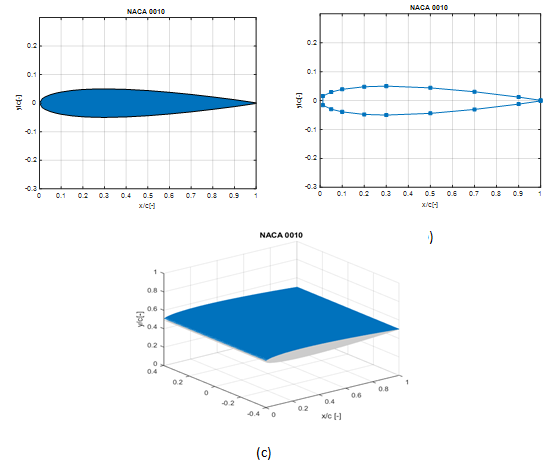Figure 1 (a) Geometry of NACA 0010 profile (b) panel approximation (c) Isometric model of NACA 0010 profile.

 Sl. No NACA Airfoil t/c, [%] Maximum camber 1 0010 10 - 2 0015 15 - 3 0024 24 - 4 4412 12 4%

Table 1 Geometrical properties of 4 digit symmetric and cambered NACA airfoils

(1)

(2)

For any NACA MPXX 4 digit airfoils, leading edge radius and trailing edge angle are function of thickness to chord ratio and given by Eq. (1) and Eq. (2).4,10 The thickness and mean line distributions for the 4 digit airfoils are expressed in terms of the t/c ratio and given by Eq. (3) to Eq. (5) respectively. It is approximated in terms of 4th order polynomial equation while the slope of camber line is obtained by differentiating the camber line function, yc and given by Eq. (6)

(3)

$\frac{{y}_{c}}{c}=\frac{M}{{\left(1-P\right)}^{2}}\left[1-2P+2P\frac{x}{c}-{\left(\frac{x}{c}\right)}^{2}\right]\right\}\frac{x}{c}\ge P$

$\frac{d{y}_{c}}{dx}=\frac{2M}{{\left(1-P\right)}^{2}}\left[P-\frac{x}{c}\right]$                 (4)

$\frac{{y}_{c}}{c}=\frac{M}{{P}^{2}}\left[2P\frac{x}{c}-{\left(\frac{x}{c}\right)}^{2}\right]$

$\frac{d{y}_{c}}{dx}=\frac{2M}{{P}^{2}}\left[P-\frac{x}{c}\right]\right\}\frac{x}{c}               (5)

(6)

Where the a0, a1 a2, a3 and a4 are the coefficients describe the shape of airfoil and its distribution of ordinates along the chord line. Airfoils with camber are denoted by letter P in Eq. (4) and Eq. (5). The extent of camber is varies along the chord length of airfoil and measured as the mean height of curvature with respect to chord line.

The most important airfoil geometric parameters are camber, thickness to chord ratio and chord length. In general, a particular flow condition is obtained by varying the angle of attack and Reynolds number. Since NACA 0010 is symmetric airfoil the camber for such airfoils is zero while thickness to chord ratio is ~10 %. In the present study the maximum thickness of 12mm was chosen with a chord length of 120mm. Wings of insects such as dragonfly have high aspect ratios and typically have chord lengths and thickness of only few mm. NACA airfoils, General Aviation(GAW) and Eppler series airfoils are recommended for comparing their aerodynamic performance with corrugated profiles at various flow configurations.5,11–13

Figure 2 is the illustration of airfoil geometric shape parameters with its leading edge curvature showing roundness of nose. Airfoils can be identified using parameters such as number of digits in series, leading edge radius, and trailing edge angle between sloping surfaces of profiles. The thickness and mean line distributions for the 4 digit symmetric and cambered airfoils are expressed in terms of the thickness to chord ratio. The series of polynomials that represent geometry of NACA 4 digit airfoils are given in text.2,10,14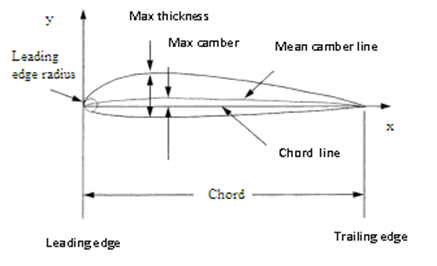Figure 2 Illustration of airfoil shape parameters.10

Panel method

For modeling 2D potential flows over streamline or bluff bodies involves solving Laplace equation to determine the velocity field. Panel methods are suitable for potential flows which involve the superposition of source or a doublet in uniform distributed flow. But they do not tend to predict accurate values of pressure and velocity fields for compressible flows over objects with complex shapes. Basic panel methods were developed by Hess and Smith at Douglas aircraft for aircraft industry.14–16 These methods were intended for analyzing the steady incompressible 2D lifting flows and model flow field by distributing source strength as nodes that are treated as singularities and having finite vortex strength centered on each panel of the surface. A source is point in which the fluid moves radially outward in field at uniform rate while for a sink fluid moves radially inwards at same uniform rate, m2/s. Each source or sink has specific strength, K, and vortex strength denoted by circulation, Г. Simple 2D uniform lifting flows14,17,18 can be described using the stream line and velocity potential functions as Eq. (7) and Eq. (8)

$\psi =U\left(ycos\alpha -xsin\alpha \right)$      (7)

$\varnothing =U\left(xcos\alpha +ysin\alpha \right)$      (8)

In a uniform flow, the stream function and velocity potential functions can be described using the Eq. (9.1) and Eq. (9.2)

(9.1)

(9.2)

Where, C and D are constants obtained after integrating the uniform flows in x and y directions in a cartesian coordinate scale. For uniform lifting flows the point source or sink is superimposed with vortex strength distributed over the geometry14 The stream function and velocity potential can be expressed in terms of the velocity components as in Eq. (10) and Eq. (11)

$u=\frac{\partial \varnothing }{\partial x}=Ucos\propto$      (10)

(11)

Where, u and v the velocity components of the free stream velocity U. The airfoil geometry is discretized into finite number of panels representing the surface. The panels are shown by series of straight line segments to construct 2D airfoil surface.14 Numbering of end points or nodes of the panels is done from 1 to N. The center point of each panel is chosen as collocation points. The impermeable periodic boundary condition of no flow orthogonal to surface is applied to every panel. Panels are defined with unit normal and tangential vectors , ,.Velocity vector, denoted by are estimated by considering the two panels, i and j representing surface of airfoil, the source on the panel j which induce a velocity on panel i. The perpendicular and tangential velocity components to the surface at the point i, are given by Eq. (12) and Eq. (13). These quantities represent the source strength on panel j and expressed mathematically as

${v}_{ij}.\stackrel{^}{n}={\sigma }_{j}{N}_{ij}$      (12)

${v}_{ij}.\stackrel{^}{t}={\sigma }_{j}{T}_{ij}$      (13)

Where ${N}_{ij}$  and  are known as normal and tangential influence coefficients. It must be noted that the velocity components induced due to source and vortex distribution at a point centered on panel i relative to panel j or point in flow field can be expressed mathematically using Eq. (14) to Eq. (16)

(14)

(15)

(16)

The surfaces represented by the panels are rectangular or curvilinear areas upon which the conditions represented by Eq. (12) to Eq. (16) are applied. The normal and tangential velocity vectors at each of collocation points consist of source and circulation strengths, which are induced due to free stream velocity, U on each panel. Together it forms a system of linear algebraic equations which can be solved for N unknown source and circulation strengths, ${\text{σ}}_{i}$ , using the influence coefficients. Mathematically it can be written in matrix form as in Eq. (17)

(17)

Along with Kutta condition explained later in this section, the matrix M contains N+1 x N+1 equations representing the ${N}_{ij}$ and , known as normal and tangential influence coefficients, A is column matrix of N elements, containing the unknown source and circulation strengths, B is the column matrix of N elements of unit normal velocity vectors. To solve for the unknown source and vortex strengths matrix inversion procedure is followed. The resultant tangential velocity vector is determined using the influence coefficients, known values of source and circulation strengths as in Eq. (18), U is the free stream velocity in m/s over the airfoil. The impermeability boundary condition is given by Eq. (19) and applied on every panel of airfoil surface.

$\sum _{j=1}^{N}{\sigma }_{j}{T}_{i,j}+\gamma {T}_{i,N+1}+\stackrel{\to }{U}×{\stackrel{^}{t}}_{i}={v}_{{t}_{i}}$        (18)

$\sum _{j=1}^{N}{\sigma }_{j}{N}_{ij}+\gamma {N}_{i,N+1}+\stackrel{\to }{U}\cdot {\stackrel{^}{n}}_{i}=0$        (19)

The pressure acting at any collocation point i on panel surface can be expressed in non–dimensional form as in Eq. (20)

(20)

Hence the influence coefficients are important for panel method in order to determine the pressure distribution over the surface of any given airfoil. It can be noted that trailing edge represents a unique condition for the airfoil. For 2D panel method, one of the following criteria14 can be used for airfoils with finite trailing edge thickness. Firstly, the streamlines near the trailing edge must be aligned in a direction which bisects the trailing edge angle. Secondly, the velocity magnitudes on the upper and lower surfaces near the trailing edge of airfoil approach the same limiting values. The trailing edge angle is modeled as the stagnation point for finite value of trailing edge angle hence the source strength must be zero at the trailing edge. This is otherwise known as Kutta condition or trailing edge boundary condition and essential for solving the matrix system of equations represented in discrete algebraic form given by Eq. (21)

$\sum _{j=1}^{N}{\sigma }_{j}{T}_{i,j}+\gamma {T}_{i,N+1}+\stackrel{\to }{U}×{\stackrel{^}{t}}_{i}=-\left(\sum _{j=1}^{N}{\sigma }_{j}{T}_{i,j}+\gamma {T}_{i,N+1}+\stackrel{\to }{U}×{\stackrel{^}{t}}_{i}\right)$       (21)

In the present method 2nd condition has been applied to evaluate the velocity vectors near trailing edge. So, the resulting tangential velocity vector is obtained by adding the known source and circulation strengths with its influence coefficients for each panel in order to calculate the pressure coefficient distribution over the entire airfoil surface. The number of panel used for simulation is 99 while the maximum panel angle between any two panels is fixed at 70. It must be noted that surface pressure coefficient can also be found from wind tunnel measurements using Eq. (22)

(22)

Where P – stagnation pressure, P is the static pressure for ambient conditions.

The flow chart depicting the panel method implemented in MATLAB software version 2019 b is shown in Figure 3.Figure 3 Computational routine of 2D panel method implemented in MATLAB (adapted from13,15)

Joukowski mapping method

A Jukouwski airfoil is obtained by the conformal transformation of cylinder of finite radius using complex mapping functions in complex plane known as ξ–η plane, ζ =ξ+iη. The conformal parameters for the Jukouwski airfoil involve angle of attack, α, thickness and camber (β). Fig. 4 shows the Jukouwski airfoil obtained using the mapping of cylinder geometry in four steps. Step 1 is solution to the potential flow in the z’’–plane containing the complex potential functions representing the uniform stream, U m/s past a cylinder, a doublet at origin, and also the circulation strength Γ. Step 2 is introducing the angle of incidence, α, by rotating the axes by an angle in the z’–plane. Step 3 determines the thickness and camber of the airfoil shape by shifting the center of circle through a distance by a in z–plane, x+iy. Step 4 involves the final Jukouwski mapping of the circle in z–plane into the airfoil shape in the ξ–η plane. Most of the Jukouwski airfoils are characterized using the parameter, β known as camber, ratio R/a known as thickness. The surface in the z’’–plane is circle (cylinder) and expressed mathematical function as Eq. (23)

(23)

Similarly after adding angle of attack, α, the solution to the flow in z–plane is given by Eq. (24)

(24)

where . The flow in z–plane after the displacement of cylinder by distance a is given by Eq. (25) as

(25)

The final step of jukouwski mapping function as in Eq. (26) represents Jukouwski airfoil in the ξ–plane and given by Eq. (27)

(26)

(27)

Where . The parameter R/a, determines the thickness of the airfoil and β is the camber. Further, it must be noted that flow variables, free stream velocity and its components, source and circulation strengths are solved in the complex z–plane using the inverse transformation method.17,19 It involves solving for the roots of quadratic function while the pressure coefficient, Cp is calculated by taking the first derivative for the complex potential function, W represented by uniform free stream flow, source and sink and circulation strength in ζ–plane (ξ+iη) as given by Eq. (28) to Eq. (30)

(28)

(29)

${C}_{p}=1-{\left(\frac{q}{U}\right)}^{2}$       (30)

Where $k=\Gamma /2\pi =–2UR.sin\left(\alpha +\beta \right)$ , $\Gamma$ is the circulation. k is the vortex strength.

# Results and discussion

In the panel method implementation, first step involves reading airfoil geometry data as input and the angle of attack defined between –20 to 100. In the next step the geometry is approximated using several panels. The number of panels representing the NACA 0010 airfoil is 99 in the simulation and trailing edge angle of 3o. In the present study, MATLAB 2019 b software was used for the flow simulation on a 2GB RAM workstation. This allows the flow to be modeled accurately within 5 % of the results predicted from other computational studies.20 The surface pressure coefficient, Cp is computed along chord length of the airfoil and for varying angles of attack, –20 to 100 as shown in Figure 5 (a) at Reynolds number of 3x105, 5x105 and 1x106. It can be observed from Figure 5(a) that for Reynolds number of 3x105 the Cp values reach a maximum of 7.6 at 10o angles of attack. However as the Reynolds number is increased to 1x106 there is shift in the peak value of Cp from 7 to 9. Since the magnitude of surface velocity on airfoil varies along its chord length the Cp values tend to reach high where the velocity is low. Further, from Figure 5(b) it can be seen that magnitude of Cp vectors are plotted at AOA of 10o along the surface airfoil for all three Reynolds numbers. The Cp values approach to higher values near leading edge stagnation point where the velocity remains zero. This suggests that the pressure gradient is favorable for which flow remains attached to boundary of airfoil between 0 and 10% chord length. However, for a given Reynolds number and at stall angle of attack, the pressure gradient becomes adverse between the upper and lower surfaces and therefore Cp vectors at trailing edge of airfoil are non–zeros for which flow is expected to become turbulent. At low angle of attack, the pressure coefficient, Cp does not vary significantly at different Reynolds numbers, and particularly for Re value of 1x 106 (1 million). It must be noted that aerodynamic flow separation on airfoils beyond stall angle of attack, using panel method cannot be modeled accurately due to numerical convergence problems. Hence it does not predict the aerodynamic flow characteristics on airfoil accurately. The present panel method has been validated using the results obtained from the inverse design method, Hess and Smith method and analytical results of Petrucci, et al.20 shown in Figure 11. Petrucci20 used vortex panel method to verify the inverse design algorithm for the incompressible flows on a conformal mapped symmetric Jukouwski airfoil having 10% thickness.20Also a source panel method implementation by Petrucci, et al20 showed geometric convergence problems for cambered airfoils. In his study, inverse design method was adopted in two steps. Firstly, flow calculation step was performed to evaluate new vortex distribution and proceed to find the induced normal velocity distribution. Secondly, geometric marching step was performed, which was essentially a modified version of leakage model by Murugesan and Railly.21 In this step, for a given panel slope angle a fictiuous vortex distribution is calculated by taking difference between required and calculated induced normal velocities at panel nodes. This step is repeated by changing panel slope angles till the final geometric shape is achieved in a closed form. In this process several iterations were required for geometric convergence in case of cambered airfoils in contrast to symmetric airfoils for different flow conditions. But in the present panel method geometric convergence was not implemented instead airfoil geometry is approximated using finite number of panels with a constant slope angle less than 15o between any two panels. As mentioned earlier, number of panels used in simulation was fixed to 99 for the flow conditions considered.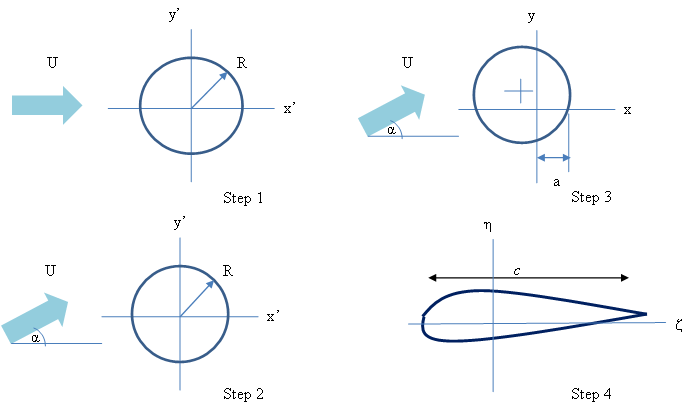Figure 4 (a)z’’ plane   (b) z’-plane   (c) z-plane     (d) ξ-η plane3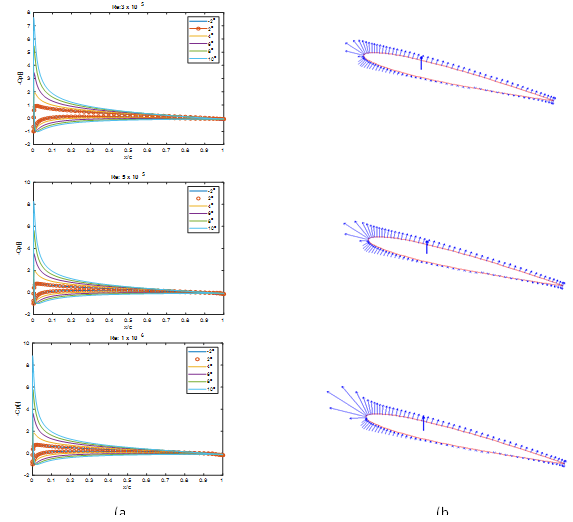Figure 5 Surface pressure coefficient distribution of NACA 0010 profile at (a) Re – 3 x 105, 5 x 105, 1 x 106 from  -2o, to 10o degree angle of attack. (b) Pressure coefficient vectors at 10o angle of attack.

From Figure 6(a) the Cp values for tripped NACA 0010 airfoil shows a higher leading edge suction peak up to 8o AOA at each of Reynolds numbers. Beyond 8o AOA, the pressure peak reduces nearly by 4% at Re – 3x105. However, this is not observed for Re–5x105 and Re–1x106. The trip location for the airfoil was chosen between 0 and 1% x/c. As mentioned before Figure 5(b) shows the Cp along with center of pressure for un–tripped NACA 0010, similarly Figure 6(b) shows the Cp vectors on the airfoil surface along with location for center of pressure for tripped NACA 0010. It can be seen that for un–tripped NACA 0010, at low Reynolds number, the pressure center is fixed and does not change along the chord length. However, for tripped NACA 0010 the location of pressure center occurs closer towards the leading edge but as the Reynolds number is increased it shifts towards downstream along the chord. Hence, pressure center location is important for the stability analysis of a pitching airfoil for aircraft wings. Figure 7(a) shows the lift coefficient between –2o and 16o AOA. The maximum lift coefficient (CLmax) increases with increase in Reynolds number. It can be seen that slope of the lift curve for un–tripped NACA 0010 is linear between 0o and 10o AOA and does not vary significantly till 10o AOA. Beyond 10o AOA the lift coefficient becomes non–linear due to the onset of stall. The CLmax for NACA 0010 at for Re–3x105 is 1.05 while for 5x105 and 1x106 it is 1.1 and 1.23 respectively. Similarly from Figure 7(b) the drag coefficient is very low between 0 and 10o AOA but increases significantly when stall angle is approached. This implies an increase of 17% and 8.5% increase in CLmax and CDmax respectively. According to13,22 the increase in pressure drag is caused due to turbulent boundary layer flow separation on suction side of airfoil. It can be noted that for finite high aspect ratio of an airfoil, tip vortices are shed quickly from trailing edge than wings with low aspect ratio. The tip vortices formed cause downwash and reduce the effective lift to drag ratios on wing. Tip vortices are also observed in case of wind turbine rotors due to which the energy losses increase when operating as an array.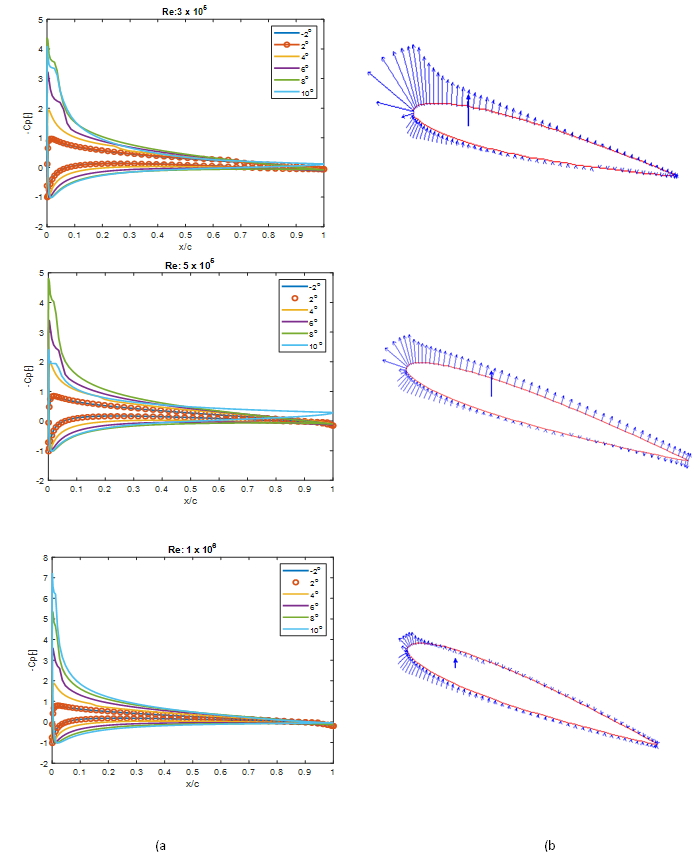Figure 6 Surface pressure coefficient distribution of NACA 0010 profile tripped at 5% chord from leading edge on suction side at (a) Re – 3 x 105, 5 x 105, 1 x 106 from  -2o, to 10o degree angle of attack. (b) Pressure coefficient vectors at 10o angle of attack.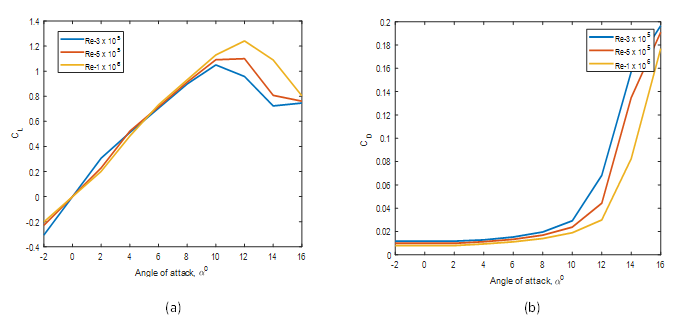Figure 7 Coefficient of lift and drag for NACA 0010 profile at Re – 3 x 105 5 x 105 and 106 between -2 to 16 degree angle of attack.

From Figure 8 the slope of the glide ratio for all three Reynolds numbers remains linear between –2o and 4o AOA. For AOA greater than 4o, the glide ratio becomes non–linear and reach peak values between 7o and 10o AOA. Evidently the height of CL/CD ratio increases with increasing Reynolds number. An increase of 45% lift to drag ratio are observed when the Reynolds number varied from 3x105 to 1x106.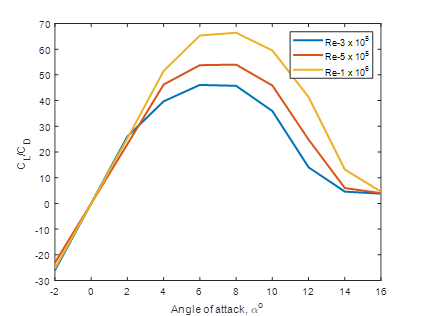Figure 8 Glide ratio or CL/CD for NACA 0010 profile at Re – 3 x 105, 5 x 105 and 106 between -2 to 16 degree angle of attack.

Similarly for Figure 9(a) and Figure 9(b) the lift and drag coefficients for the tripped NACA 0010 airfoil show similar trends as observed in un–tripped airfoil. The drag coefficient beyond 12o AOA reduced at Reynolds number of 1x106. This shows that boundary layer or pressure drag is reduced on the suction side of airfoil due to reduction of boundary layer thickness and increase in momentum or kinetic energy transfer from the free stream flow regions to the boundary layer.Figure 9 Coefficient of lift and drag for NACA 0010 profile tripped at 5% chord from leading edge on suction side at Re – 3 x 105 5 x 105 and 106 between -2 to 16 degree angle of attack.

From Figure 10 it can be seen that maximum lift to drag ratio for tripped NACA 0010 airfoil reach nearly 70 and show an increase of 40% in aerodynamic performance. Compared to tripped airfoil, the increase of 5% in aerodynamic performance is clearly visible.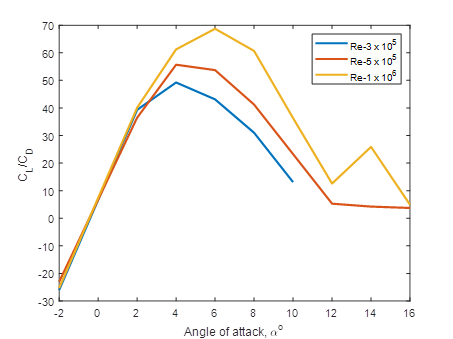Figure 10 Glide ratio or CL/CD for NACA 0010 profile tripped at 5% chord from leading edge on suction side for Re – 3 x 105 , 5 x 105 and 106 between -2 to 16 degree angle of attack.

In the study of Petrucci20 the results of the pressure coefficient obtained from inverse design algorithm were also compared with analytical results of the boundary integral method that includes boundary layer thickness and viscous effects. The pressure peaks near the leading edge are under predicted in all inverse design methodologies. In Figure 11 the results for Cp, obtained using inverse design method of Petrucci20 are found to under predict relative to those obtained from present panel method however, they agree well within 1% of the results from analytical or boundary integral formulation. The results of Hess & Smith method obtained from Petrucci 20 are in better agreement with the present 2D panel method, in terms of the pressure peaks observed at the leading edge on the upper surface of NACA 0010 airfoil and up to 80% chord length. However, between 80–100% of chord, the pressure coefficient remained gradually reducing and found to vary by ~5 % near the trailing edge section of the airfoil. Thus pressure distribution on airfoil provides essential data for determining the aerodynamic behavior of wings with complex design shapes. The aircraft wings built from airfoils have finite length and thickness with sharp or cusped trailing edge. The thickness of the trailing edge influences the pressure and velocity distribution on the wing at different angles of attack. The velocity contours for the conformal mapped symmetric jukouwski airfoil of 10% thickness are evaluated in MATLAB and shown for different angles of attack in Figure 12. A Matlab program was developed to demonstrate the similarity in results obtained for pressure coefficient and to characterize the flow around airfoil. Similarly the pressure coefficient contours are evaluated for the same flow conditions for the airfoil. Figure 13 shows the surface pressure coefficient contours at different angles of attack for the symmetric Joukowski airfoil of same chord length as that of NACA 0010 airfoil.Figure 11 Comparison of surface pressure coefficient distribution of NACA 0010 profile at Re – 3 x 105 for 40angle of attack using Hess & Smith, Analytical and Petrucci inverse design method6 with present panel method result for conformal mapped symmetric Jukouwski airfoil of thickness 10%, at 40angle of attack.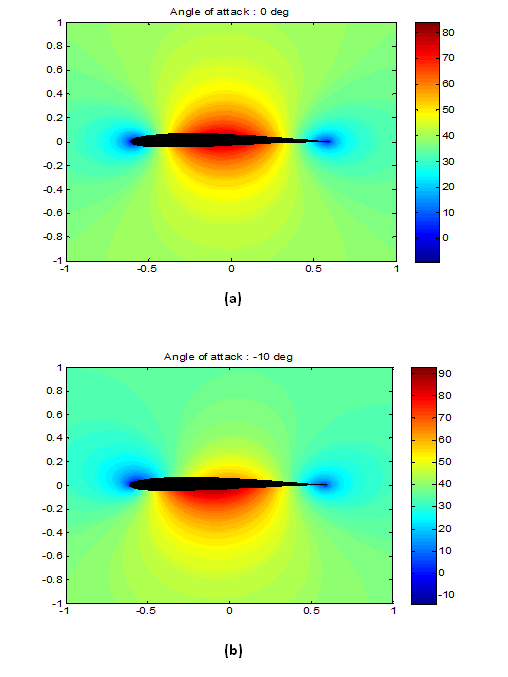Figure 12 Velocity contours for conformal mapped symmetric Joukowski airfoil at U = 38m/s (a) at -100 AOA (b) 00 AOA (c) 120 AOA (d) 160 AOA.Figure 13 Pressure coefficient contours for conformal mapped symmetric jukouwski airfoil for U= 38 m/s  (a) at -10o AOA (b) 0o AOA (c) 12o AOA (d) 16o AOA.

At 0oAOA, the velocity contours and pressure coefficient show symmetry around the airfoil chord length as shown in Figure 12(b) and Figure 13(b). As the angle of attack is varied, at –10o AOA, the velocity and pressure coefficient contours on the upper and lower surface of the airfoil are reversed as shown in Figure 12(a) and Figure 13(a). The maximum deviation in the computed results from present panel method and results obtained from, Hess and Smith, Inverse design methods for conformal mapped symmetric jukouwski airfoil are found to be ~20 % near trailing edge of airfoil. It is due to the implementation of kutta condition for the trailing edge panels required for geometry closure and ensuring smooth flow. The flow around trailing edge of any cusped airfoil is associated with finite circulation formed due to superimposition of source and vortex strengths. In aerodynamics, the strength of circulation is strong determinant of lift force on airfoil. It is proportional to lift and depend on the fluid density, viscosity and free stream velocity. For a symmetric airfoil with finite bound circulation, the leading edge and trailing edge stagnation point coincides for 0o AOA. However, for non–zero and high positive AOA the strength of circulation does not remain constant along the chord. In fact it facilitates the trailing edge vortex formation due to stall observed on the suction or low pressure surface of airfoil. It can be attributed to change in momentum along chord direction on suction and pressure sides of airfoil. Further it must be noted up to 60% normalized chord length (x/c), the pressure coefficients are found to deviate by less than 5 %. From 60% x/c to trailing edge tip, the pressure coefficients were found to agree within 1% with Hess and Smith, Inverse design and Analytical methods on both suction and pressure sides of airfoil. The sketch of the mapped airfoil, size of cylinder of radius, R = 0.3 units is shown in Figure 14. The displacement of the cylinder or circle in the real axis (x–axis) is set to 0.03 units to obtain a chord length of 1.2 units equal to ~ 120mm and thickness 10 % or ~12 mm. Figure 15(a) to Figure 15(d) shows the streamline contours around Jukouwski cylinder and its conformal mapped Jukouwski airfoil at different angles of attack for the same free stream velocity, U = 38 m/s symmetric jukouwski airfoil of 10% thickness, U = 38 m/s (a) –100 AOA (b) 00 AOA (c) 120 AOA (d) 160 AOA.23–25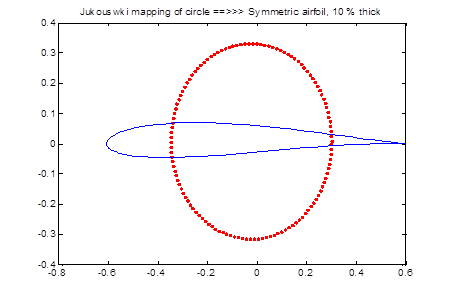Figure 14 Geometry of conformal mapped Jukouwski airfoil and cylinder of radius 0.3 units.Figure 15 Normalized streamline contours for cylinder and mapped symmetric jukouwski airfoil of 10 % thickness, U = 38 m/s (a) -100 AOA (b) 00 AOA (c) 120 AOA (d) 160 AOA.

# Conclusion

2D Panel and Jukouwski methods have been implemented on NACA0010 airfoil at moderate to high Reynolds number between –20 to 100 angles of attack. The velocity and pressure coefficient contours have been evaluated between –10o and 16o angles of attack using Jukouwski mapping and panel methods. The present numerical results were found to be close agreement with the Hess and Smith, inverse design methods applied for conformal mapped symmetric Joukouwski airfoil of comparable thickness to chord ratio. The results for surface pressure coefficient using present panel method agreed well within 4% on upper surface compared to those obtained from the inverse design, Hess and Smith results. On lower surfaces of airfoil it was found to vary by ~25% near trailing edge of airfoil. In comparison to the panel method, results from the Jukouwski mapping method predicted the pressure coefficient conservatively for the same free stream conditions. With increase in Reynolds number, lift coefficient improved by 18% when the angle of attack approached stall angle, similarly lift to drag ratios for both un–tripped and tripped airfoil conditions also increased by 40%. Hence the results of present study can be used to facilitate the design of improved airfoils for use in development of high efficiency rotors and propellers or in micro aerial vehicles.

# References

1. Eppler R. Airfoil Design and Data. Spring Verlag. 1990.
2. L J Clancy. Indian edn, Aerodynamics. Sterling Book House, New Delhi.
3. Xiao S, Chen, Z. Investigation of flow over the airfoil NACA 0010–35 with various angle of attack, 2nd international conference on mechanical, material and aerospace engineering. 2018.
4. http://web.mit.edu/drela/Public/web/xfoil/
5. Vargas A, Mittal R, Dong H. A computational study of aerodynamic performance of dragon flies wing section in gliding flight. George Washington University, Washington DC 20052. USA,  2008.
6. Craig W Somerton, Andrew Huisjen. Aerospace engineering fundamentals, ME 440. Michigan State University, USA.
7. Dwivedi YD, Bhargava V, Maddula SP, et al. Prediction of atmospheric turbulence characteristics for surface boundary layer using empirical spectrum methods. Revista Brasileira de Meteorologia. 2020;36(1).
8. Bhargava V, Samala R, Anumula C. Prediction of broadband noise from symmetric and cambered airfoils. INCAS Bulletin. 2018;11(1):39–51.
9. Naveen G, Mahato B, Bhumkar YG. Prediction of aerodynamic sound generated due to flow over cylinder performing steady rotation and rotary oscillations. The Journal of Acoustical Society of America. 2020;147(1):325.
10. Abbott E, Von Doenhoff. Theory of Wing Sections, including summary of airfoil data, Dover Publications Inc, New York.
11. Gao H, Hu H, Wang Z. Computational study of flows around Dragon fly and smooth airfoils at low Reynolds number. 46th AIAA Aerospace sciences meeting and Exhibit, Nevada, USA, 2008.
12. Sridhar V, Dwivedi YD. Effect of peak shape in bio inspired corrugated wing, International conference on advances in thermal systems. materials and design engineering. Mumbai, ATSMDE. 2017.
13. Rossi E, Colagrossi A, Oger G, et al. Multiple bifurcations of the flow over stalled airfoils when changing the Reynolds number. 2018.
14. Haughton, Carpenter, 6th edn, Aerodynamics for Engineering Students. Elsevier publications. New York.
15. Som SK, Biswas. An introduction to fluid mechanics and fluid machinery. Mc Graw Hill publications. New Delhi.
16. Hess JL. A higher order panel method for three dimensional potential flow. 7th Australasian hydraulics and fluid mechanics conference, Brisbane, Australia. 1979.
17. Jain R, Sheikh MY, Singh D, et al. Numerical Analysis of Joukowski (T = 12%) Airfoil by k–ε Turbulence Model at High Reynolds Number. In: Pandit M et al., editors. Intelligent Computing Applications for Sustainable Real–World Systems. ICSISCET 2019. Proceedings in Adaptation, Learning and Optimization, volume 13 Springer, Cham. 2020.
18. Nilavarasan T, Joshi GN, Chandel S. Aerodynamic performance characteristics of NACA 0010 cascade with Gurney flaps. International journal of turbo jet engineering. 2018.
19. Newman SJ. Joukowski Aerofoil Modeling. Technical report AFM–11/13. University of Southampton, 2011.
20. Petrucci D, Filho NM. A fast algorithm for inverse airfoil design using a transpiration model and an improved vortex panel method. Journal of Brazil society of Mechanical Science & Engineering. 2007;29(4).
21. Murugesan K, Railly JW. Pure Design Method for Aerofoils in Cascade. Journal of Mechanical Engineering Science. 11(5):454–467.
22. Da Silva D, Malatesta V. Numerical simulation of the boundary layer control on the NACA 0015 airfoil through vortex generators. Journal of aerospace technology and management. 2019;12(1).
23. Swamy Naidu NV, Maddula SP, Bhargava VN. A study of high lift aerodynamic devices on commercial aircrafts. Aviation VGTU Journals. 2020;1(3):1–14.
24. Dwivedi YD, Bhargava V, Rao PMV, et al. Aerodynamic characterization of bio inspired corrugated wings. INCAS Bulletin. 2019;11(1):1–14.
25. Benton SI, Visbal MR. Understanding abrupt leading edge separation as a mechanism for the onset of dynamic stall. AIAA aerospace sciences meeting. 2018;1–16.